## TONEMAP1

 Enhances the shadows and/or highlight regions in an image using a non-linear log or gamma function.

### EXAMPLES

(Processed With Q16 HDRI IM Compile)

 Note: Over time there have been changes to IM. Therefore, some examples now are better and some worse. So some arguments may need changing, but may not fix all the examples. Also -C sRGB or RGB will need swapping on current versions of IM.

 Example 1 -- mode=all -- variation in edge enhancement Original Displayed As JPEG (Actual EXR Image Used) (source image web site)Arguments: -m all -s 0 -h -10 -e 0 -S 2.5 Arguments: -m all -s 0 -h -10 -e 100 -S 2.5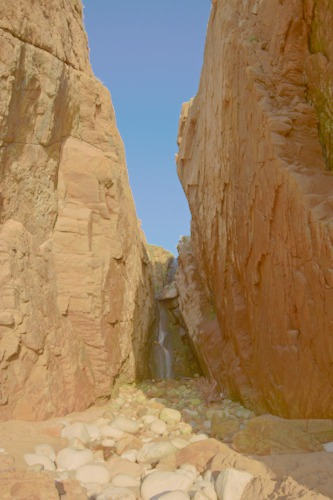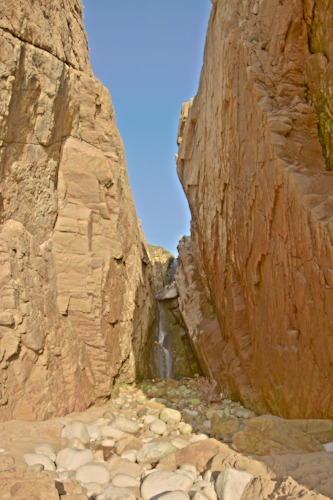Example 2 -- mode=intensity -- variation in edge enhancement Original Displayed As JPEG (Actual EXR Image Used) (source image web site)Arguments: -m intensity -s 0 -h -10 -e 0 -S 0.5 Arguments: -m intensity -s 0 -h -10 -e 100 -S 0.5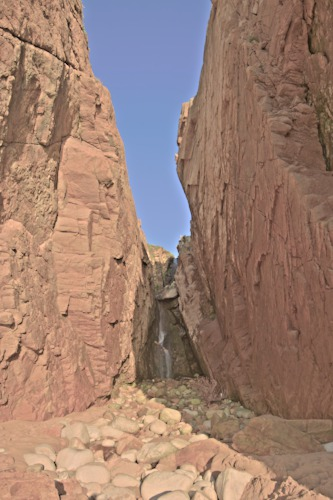Example 3 -- mode=all vs mode=intensity Original Displayed As JPEG (Actual EXR Image Used) (source image web site)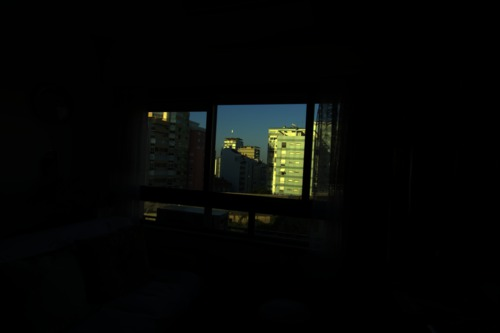Arguments: -m all -s 30 -h -10 -e 50 -S 2Arguments: -m intensity -s 30 -h -10 -e 50 -S 0.6Example 4 -- mode=all vs mode=intensity Original Displayed As JPEG (Actual EXR Image Used) (source image web site)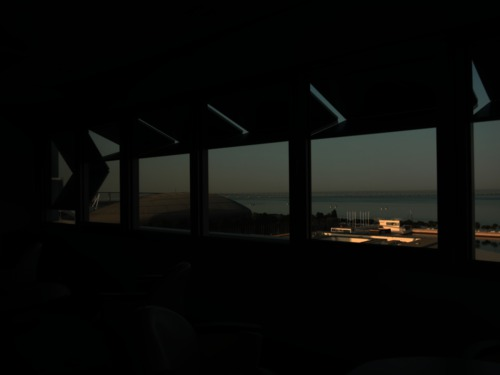Arguments: -m all -s 30 -h -10 -e 50 -S 2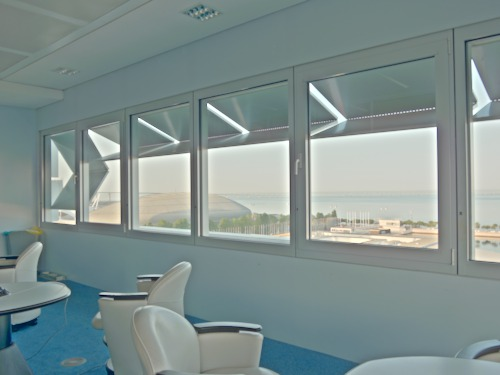Arguments: -m intensity -s 30 -h -10 -e 50 -S 0.5Example 5 -- mode=all vs mode=intensity Original Displayed As JPEG (Actual EXR Image Used) (source image web site)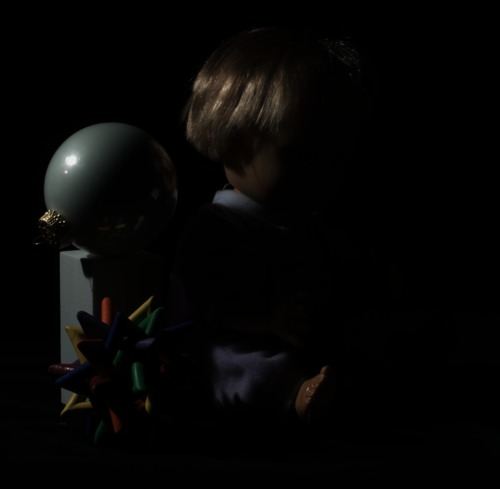Arguments: -m all -s 20 -h -10 -e 100 -S 2Arguments: -m intensity -s 20 -h -10 -e 100 -S 0.5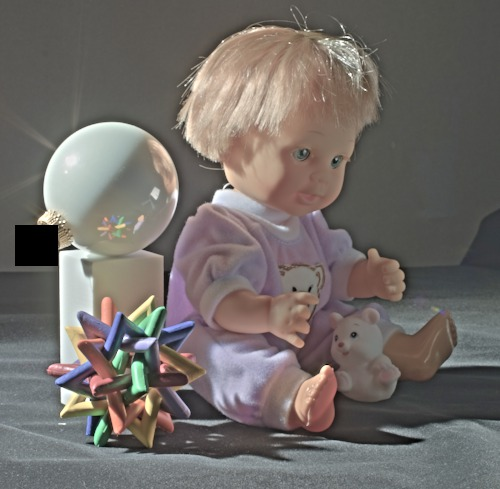Example 6 -- mode=all vs mode=intensity Original Displayed As JPEG (Actual HDR Image Used) (source image web site)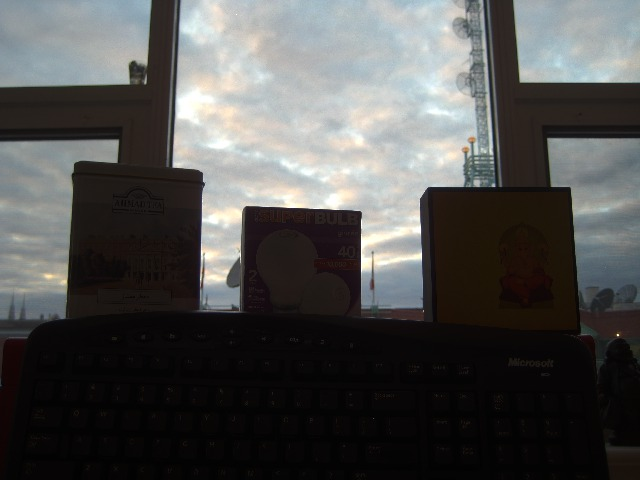Arguments: -m all -s -10 -h 0 -e 100 -S 2.5 -C sRGB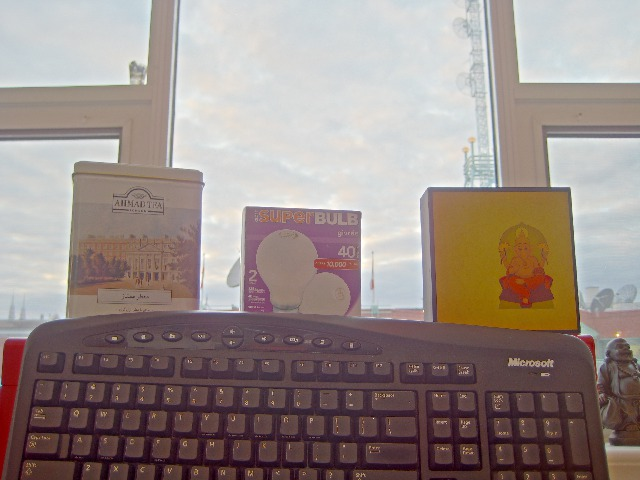Arguments: -m intensity -s -10 -h 0 -e 100 -S 0.5 -C sRGB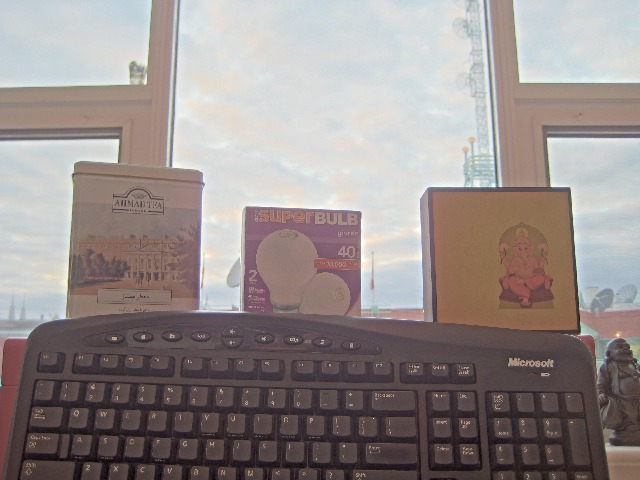Example 7 -- mode=all vs mode=intensity Original Displayed As JPEG (Actual HDR Image Used) (source image web site) (NOTE: Processed after .hdr bug fix at IM 6.7.2.0)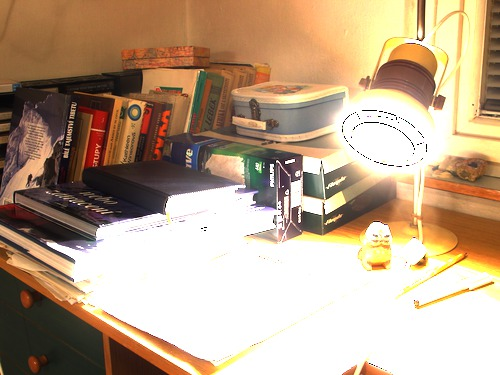Arguments: -m all -s -40 -h -40 -e 100 -S 3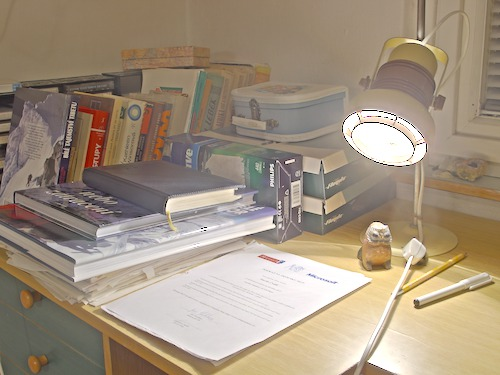Arguments: -m intensity -s -40 -h -40 -e 100 -S 0.4What the script does is as follows for mode=intensity: Converts the image to HSB or HSL colorspace and separate channels Enhance the saturation channel Apply the non-linear function to the intensity channel (B or L) Blur the enhanced intensity channel Subtract the blurred intensity channel from the non-blurred intensity channel to form an edge image Enhance the blurred intensity channel Enhance the edge image Add the edge image with the blurred image as a modified intensity image Combine the original Hue channel with the modified Saturation channel and the modified intensity channel and converts back to RGB This is equivalent to the following IM commands for mode=intensity: if [ "\$cspace" = "none" ]; then cproc="" else cproc="-set colorspace \$cspace" fi if [ "\$alevel" = "yes" ]; then aproc="-auto-level" else aproc="" fi convert -quiet -regard-warnings "\$infile" -alpha off \$cproc \$aproc +repage "\$tmpA1" if [ "\$kind" = "log" ]; then amount=`convert xc: -precision 8 -format "%[fx:10^\$amount]" info:` proc0="-evaluate log \$amount" elif [ "\$kind" = "gamma" ]; then proc0="-gamma \$amount" fi if [ "\$sat" = "1" ]; then saturation="" else saturation="-evaluate multiply \$sat" fi ssign=`convert xc: -format "%[fx:sign(\$shadows)==-1?1:0]" info:` hsign=`convert xc: -format "%[fx:sign(\$highlights)==-1?1:0]" info:` mlo=0 mhi=100 plo=0 phi=100 if [ "\$shadows" != "0" -a \$ssign -eq 1 ]; then mlo=\$((-shadows)) elif [ "\$shadows" != "0" -a \$ssign -eq 0 ]; then plo=\$shadows fi if [ "\$highlights" != "0" -a \$hsign -eq 1 ]; then phi=\$((100+highlights)) elif [ "\$highlights" != "0" -a \$hsign -eq 0 ]; then mhi=\$((100-highlights)) fi if [ \$mlo -eq 0 -a \$mhi -eq 100 ]; then proc1="" else proc1="-level \$mlo,\$mhi%" fi if [ \$plo -eq 0 -a \$phi -eq 100 ]; then proc2="" else proc2="+level \$plo,\$phi%" fi edge=`convert xc: -format "%[fx:1+\$edge/100]" info:` eproc="-evaluate multiply \$edge" convert \$tmpA1 -colorspace \$colormode -separate \ \( -clone 1 \$saturation \) \ \( -clone 2 \$proc0 \) \ \( -clone 4 -blur 0x\$blur \) \ \( -clone 4 -clone 5 +swap -compose minus -composite \$eproc \) \ \( -clone 5 \$proc1 \$proc2 \) \ \( -clone 6 -clone 7 -compose plus -composite \) \ -delete 1,2,4,5,6,7 -set colorspace \$colormode -combine -colorspace RGB \ \$outfile